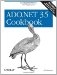# Copying Rows from One DataTable to Another

Problem

You have records in a DataTable that you need to copy to another DataTable .

Solution

Use the ImportRow( ) method of the DataTable to copy DataRow objects from one DataTable to another. Three techniques for selecting records to copy are demonstrated in the following example:

• Use the Rows property to access rows in the DataRowCollection of the DataTable using the row index.
• Use the Select( ) method of the DataTable .
• Use the RowFilter property of a DataView for the DataTable .

The sample code creates a source DataTable containing the Orders table from Northwind. A second empty target DataTable is created with the same schema. One of the three techniques, as specified by the user , is used to copy records from the source table to the target table.

The C# code is shown in Example 5-1.

Example 5-1. File: CopyRowsBetweenTablesForm.cs

```// Namespaces, variables, and constants
using System;
using System.Configuration;
using System.Data;
using System.Data.SqlClient;

// Table name constants
private const String ORDERS_TABLE = "Orders";

// Field name constants
private const String ORDERID_FIELD = "OrderID";

// . . .

// Fill the source table with schema and data.
ConfigurationSettings.AppSettings["Sql_ConnectString"]);
DataTable dt = new DataTable(ORDERS_TABLE);
da.FillSchema(dt, SchemaType.Source);
da.Fill(dt);

// Clone the schema to the copy table.
DataTable dtCopy = dt.Clone( );

{
// Use ImportRow method to import the first three rows.
for (int i = 0; i < 3; i++)
{
dtCopy.ImportRow(dt.Rows[i]);
}
}
{
// Copy using result of Select( ) method.
foreach(DataRow row in dt.Select(ORDERID_FIELD + " <= 10300"))
{
dtCopy.ImportRow(row);
}
}
{
// Copy using result of filtered DataView.
DataView categoryView = dt.DefaultView;
categoryView.RowFilter = ORDERID_FIELD + " >= 10300 AND " +
ORDERID_FIELD + " < 10400";
for (int i = 0; i < categoryView.Count; i++)
{
dtCopy.ImportRow(categoryView[i].Row);
}
}

// Bind the default view of the copy table to the grid.
dataGrid.DataSource = dtCopy.DefaultView;
```

Discussion

Rows can be copied between tables using the ImportRow( ) methods of the DataTable object. The ImportRow( ) method requires that both the source and destination table have the same structure. In this example, the Clone( ) method of the DataTable creates a table with identical structure, but any technique that creates a table with an identical structure can be used.

Additionally, the Copy( ) method of the DataTable object can create a new DataTable having the same structure and data as the original as shown in the following code sample:

```// Create the source table.
DataTable dtSource = new DataTable("Source");

// . . . Fill the source table with data.

// Create the destination table and copy the source table.
DataTable dtDest = new DataTable("Dest");
dtDest = dtSource.Copy( );
```